Home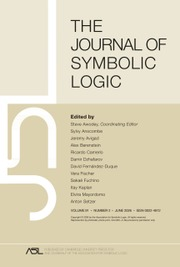The Journal of Symbolic Logic

# PREDICATIVITY THROUGH TRANSFINITE REFLECTION

Published online by Cambridge University Press:  08 September 2017

Corresponding

## Abstract

Let T be a second-order arithmetical theory, Λ a well-order, λ < Λ and X ⊆ ℕ. We use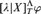$[\lambda |X]_T^{\rm{\Lambda }}\varphi$ as a formalization of “φ is provable from T and an oracle for the set X, using ω-rules of nesting depth at most λ”.

For a set of formulas Γ, define predicative oracle reflection for T over Γ (Pred–O–RFNΓ(T)) to be the schema that asserts that, if X ⊆ ℕ, Λ is a well-order and φ ∈ Γ, then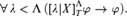$$\forall \,\lambda < {\rm{\Lambda }}\,([\lambda |X]_T^{\rm{\Lambda }}\varphi \to \varphi ).$$

In particular, define predicative oracle consistency (Pred–O–Cons(T)) as Pred–O–RFN{0=1}(T).

Our main result is as follows. Let ATR0 be the second-order theory of Arithmetical Transfinite Recursion,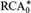${\rm{RCA}}_0^{\rm{*}}$ be Weakened Recursive Comprehension and ACA be Arithmetical Comprehension with Full Induction. Then,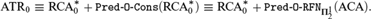$${\rm{ATR}}_0 \equiv {\rm{RCA}}_0^{\rm{*}} + {\rm{Pred - O - Cons\ }}\left( {{\rm{RCA}}_0^{\rm{*}} } \right) \equiv {\rm{RCA}}_0^{\rm{*}} + \,{\rm{Pred - O - Cons\ }}\left( {{\rm{RCA}}_0^{\rm{*}} } \right) \equiv {\rm{RCA}}_0^{\rm{*}} + \,{\rm{Pred - O - RFN}}\,_{{\bf{\Pi }}_2^1 } \left( {{\rm{ACA}}} \right).$$

We may even replace${\rm{RCA}}_0^{\rm{*}}$ by the weaker ECA0, the second-order analogue of Elementary Arithmetic.

Thus we characterize ATR0, a theory often considered to embody Predicative Reductionism, in terms of strong reflection and consistency principles.

## Keywords

Type
Articles
Information
The Journal of Symbolic Logic , September 2017 , pp. 787 - 808

## Access options

Get access to the full version of this content by using one of the access options below.

## References

Beeson, M. and Ščedrov, A., Church’s thesis, continuity, and set theory, this Journal, vol. 49 (1984), no. 2, pp. 630–643.
Beklemishev, L. D., Induction rules, reflection principles, and provably recursive functions. Annals of Pure and Applied Logic, vol. 85 (1997), pp. 193242.CrossRefGoogle Scholar
Beklemishev, L. D., Provability algebras and proof-theoretic ordinals, I. Annals of Pure and Applied Logic, vol. 128 (2004), pp. 103124.CrossRefGoogle Scholar
Beklemishev, L. D., Veblen hierarchy in the context of provability algebras, Logic, Methodology and Philosophy of Science, Proceedings of the Twelfth International Congress (Hájek, P., Valdés-Villanueva, L., and Westerståhl, D., editors), Kings College Publications, London, 2005, pp. 6578.Google Scholar
Beklemishev, L. D., Reflection principles and provability algebras in formal arithmetic. Russian Mathematical Surveys, vol. 60 (2005), pp. 197268.CrossRefGoogle Scholar
Beklemishev, L. D., The Worm principle, Logic Colloquium 2002 (Chatzidakis, Z., Koepke, P., and Pohlers, W., editors), Lecture Notes in Logic 27, ASL Publications, 2006, pp. 7595.Google Scholar
Beklemishev, L. D., On the reduction property for GLP-algebras. Doklady: Mathematics, vol. 472 (2017), no. 4.Google Scholar
Beklemishev, L. D., Fernández-Duque, D., and Joosten, J. J., On provability logics with linearly ordered modalities. Studia Logica, vol. 102 (2014), pp. 541566.CrossRefGoogle Scholar
Beklemishev, L. D. and Onoprienko, A. A., On some slowly terminating term rewriting systems. Sbornik: Mathematics, vol. 206 (2015), no. 9, pp. 11731190.CrossRefGoogle Scholar
Boolos, G. S., The Logic of Provability, Cambridge University Press, Cambridge, 1993.Google Scholar
Cordón-Franco, A., Fernández-Margarit, A., and Lara-Martín, F. F., Fragments of Arithmetic and true sentences. Mathematical Logic Quarterly, vol. 51 (2005), pp. 313328.CrossRefGoogle Scholar
Feferman, S., Systems of predicative analysis, this Journal, vol. 29 (1964), pp. 1–30.
Feferman, S., Systems of predicative analysis II, this JOURNAL, vol. 33 (1968), pp. 193–220.
Fernández-Duque, D., The polytopologies of transfinite provability logic. Archive for Mathematical Logic, vol. 53 (2014), no. 3–4, pp. 385431.CrossRefGoogle Scholar
Fernández-Duque, D. and Joosten, J. J., Models of transfinite provability logics, this Journal, vol. 78 (2013), no. 2, pp. 543–561.
Fernández-Duque, D. and Joosten, J. J., The omega-rule interpretation of transfinite provability logic, (2013), arXiv, vol. 1205.2036 [math.LO].
Fernández-Duque, D. and Joosten, J. J., Well-orders in the transfinite Japaridze algebra. Logic Journal of the Interest Group in Pure and Applied Logic, vol. 22 (2014), no. 6, pp. 933963.Google Scholar
Hájek, P. and Pudlák, P., Metamathematics of First Order Arithmetic, Springer-Verlag, Berlin, Heidelberg, New York, 1993.CrossRefGoogle Scholar
Hirst, J. L., A survey of the reverse mathematics of ordinal arithmetic, Reverse mMathematics 2001, Lecture Notes in Logic, vol. 21, Peters, A. K., Natick, MA, 2005, pp. 222234.Google Scholar
Ignatiev, K. N., On strong provability predicates and the associated modal logics, this Journal, vol. 58 (1993), pp. 249–290.
Japaridze, G., The polymodal provability logic, Intensional Logics and Logical Structure of Theories: Material from the Fourth Soviet-Finnish Symposium on Logic, Metsniereba, Telaviv, 1988, pp. 1648, In Russian.Google Scholar
Joosten, J. J., .${\rm{\Pi }}_1^0$ -ordinal analysis beyond first-order arithmetic. Mathematical Communications, vol. 18 (2013), pp. 109121.Google Scholar
Kreisel, G. and Lévy, A., Reflection principles and their use for establishing the complexity of axiomatic systems. Zeitschrift für mathematische Logik und Grundlagen der Mathematik, vol. 14 (1968), pp. 97142.CrossRefGoogle Scholar
Leivant, D., The optimality of induction as an axiomatization of arithmetic, this Journal, vol. 48 (1983), pp. 182–184.
Schmerl, U. R., A fine structure generated by reflection formulas over primitive recursive arithmetic, Logic Colloquium ’78 (Mons, 1978) (Boffa, M., Dalen, D., and Mcaloon, K., editors), Studies in Logic and the Foundations of Mathematics, vol. 97, North-Holland, Amsterdam, 1979, pp. 335350.Google Scholar
Simpson, S. G., Friedman’s research on subsystems of second-order arithmetic, Harvey Friedman’s Research in the Foundations of Mathematics (Harrington, L., Morley, M., Ščedrov, A, and Simpson, S. G., editors), North-Holland, Amsterdam, 1985, pp. 137159.CrossRefGoogle Scholar
Simpson, S. G., Subsystems of Second Order Arithmetic, Cambridge University Press, New York, 2009.CrossRefGoogle Scholar
Simpson, S. G. and Smith, R. L., Factorization of polynomials and${\rm{\Sigma }}_1^0$ induction. Annals of Pure and Applied Logic, vol. 31 (1986), pp. 289306.CrossRefGoogle Scholar
Tait, W., Finitism. Journal of Philosophy, vol. 78 (1981), pp. 524546.CrossRefGoogle Scholar

### Full text views

Full text views reflects PDF downloads, PDFs sent to Google Drive, Dropbox and Kindle and HTML full text views.

Total number of HTML views: 9
Total number of PDF views: 79 *
View data table for this chart

* Views captured on Cambridge Core between 08th September 2017 - 1st December 2020. This data will be updated every 24 hours.

Hostname: page-component-79f79cbf67-b9sxz Total loading time: 12.442 Render date: 2020-12-01T21:42:47.517Z Query parameters: { "hasAccess": "0", "openAccess": "0", "isLogged": "0", "lang": "en" } Feature Flags last update: Tue Dec 01 2020 21:06:11 GMT+0000 (Coordinated Universal Time) Feature Flags: { "metrics": true, "metricsAbstractViews": false, "peerReview": true, "crossMark": true, "comments": true, "relatedCommentaries": true, "subject": true, "clr": false, "languageSwitch": true }

# Send article to Kindle

Note you can select to send to either the @free.kindle.com or @kindle.com variations. ‘@free.kindle.com’ emails are free but can only be sent to your device when it is connected to wi-fi. ‘@kindle.com’ emails can be delivered even when you are not connected to wi-fi, but note that service fees apply.

Find out more about the Kindle Personal Document Service.

PREDICATIVITY THROUGH TRANSFINITE REFLECTION
Available formats
×

# Send article to Dropbox

To send this article to your Dropbox account, please select one or more formats and confirm that you agree to abide by our usage policies. If this is the first time you use this feature, you will be asked to authorise Cambridge Core to connect with your <service> account. Find out more about sending content to Dropbox.

PREDICATIVITY THROUGH TRANSFINITE REFLECTION
Available formats
×

# Send article to Google Drive

To send this article to your Google Drive account, please select one or more formats and confirm that you agree to abide by our usage policies. If this is the first time you use this feature, you will be asked to authorise Cambridge Core to connect with your <service> account. Find out more about sending content to Google Drive.

PREDICATIVITY THROUGH TRANSFINITE REFLECTION
Available formats
×
×